# Data Analysis

## Decision trees1

1. Some materials are taken from machine learning course of Victor Kitov

# Let's recall previous lecture¶

• Metric methods: Nearest Centroid, K Nearest Neighbours
• Work both for classification and regression
• Lazy learning - simply remember training dataset
• No parameters - only hyper-parameters
• Cluster hypothesis - the core of metric methods
• Similarity measures and distances: euclidean, cosine, edit-distance, Jaccard similarity, etc...
• Feature scaling is important!
• Various modifications:
• weighted domain
• Get ready to face with
• Curse of dimentionality (about that in the next lectures)
• Slow prediction speed

# Intuition¶

## Intuition 1¶

• A perfumery company developed a new unisex parfume
• To find their key segments it they run open world testing
• Each respondent leaves
• responce if she likes it or not (+1|-1)
• Gender
• Age
• Education
• Current career
• Have domestic animals
• etc..

## Intuition 1¶

In the end the description of the segments could look like this

• [Gender = F][Age > 21][Age <= 25][Education = Higher][Have domestic animals = No] - like in 82% of cases
• [Gender = M][Age > 25][Age <= 30][Current Career = Manager] - don't like in 75% of cases
• ...

## Intuition 2¶

• You are going to take a loan god, please, no to buy something expensive, and provide your application form
• Bank employee is checking it accoring to some rules like:
1. Current bank account > 200k rubles. - go to step 2, otherwise 3
2. Duration < 30 months - go to step 4, otherwise REJECT
3. Current employment > 1 year - ACCEPT, otherwise REJECT
4. ...

## Intuition 2¶## Intuition 3¶## Intuition 4¶## Intuition 4¶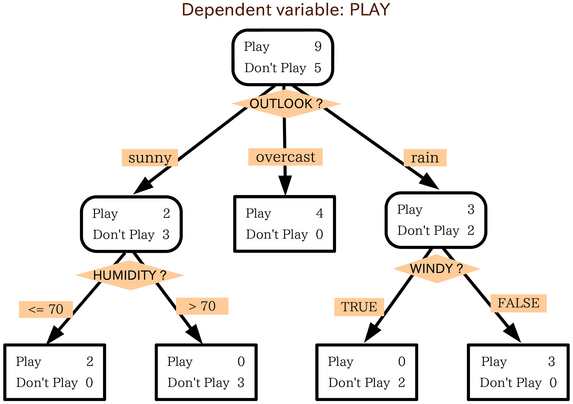## Definition of decision tree¶

• Prediction is performed by tree $T$ (directed, connected, acyclic graph)

• Node types

1. A root node
2. Internal nodes, each having $\ge2$ child nodes
3. Terminal nodes (leaves), which do not have child nodes but have associated prediction values

## Definition of decision tree¶

• for each non-terminal node $t$ a check-function $Q_{t}(x)$ is associated
• for each edge $r_{t}(1),...r_{t}(K_{t})$ a set of values of check-function $Q_{t}(x)$ is associated: $S_{t}(1),...S_{t}(K_{t})$ such that:
• $\bigcup_{k}S_{t}(k)=range[Q_{t}]$
• $S_{t}(i)\cap S_{t}(j)=\emptyset$ $\forall i\ne j$

## Prediction process¶

• Prediction is easy if we have already constructed a tree
• Prediction process for tree $T$:

• $t=root(T)$
• while $t$ is not a terminal node:

• calculate $Q_{t}(x)$
• determine $j$ such that $Q_{t}(x)\in S_{t}(j)$
• follow edge $r_{t}(j)$ to $j$-th child node: $t=\tilde{t}_{j}$
• return prediction, associated with leaf $t$.

## Specification of decision tree¶

• To define a decision tree one needs to specify:
• the check-function: $Q_{t}(x)$
• the splitting criterion: $K_{t}$ and $S_{t}(1),...S_{t}(K_{t})$
• the termination criteria (when node is defined as a terminal node)
• the predicted value for each leaf node.

## Generalized decision tree algorithm¶

{python}
1. function decision_tree(X, y):

2.    if termination_criterion(X, y) == True:

3.        S = create_leaf_with_prediction(y)

4.    else:

5.        S = create_node()
6.        (X_1, y_1) .. (X_L, y_L) = best_split(X, y)

7.        for i in 1..L:
8.            C = decision_tree(X_i, y_i)
9.            connect_nodes(S, C)
10.   return S

# Splitting rules¶

## Possible definitions of splitting rules¶

• $Q_{t}(x)=x^{i(t)}$, where $S_{t}(j)=v_{j}$, where $v_{1},...v_{K}$ are unique values of feature $x^{i(t)}$.
• $S_{t}(1)=\{x^{i(t)}\le h_{t}\},\,S_{t}(2)=\{x^{i(t)}>h_{t}\}$
• $S_{t}(j)=\{h_{j}<x^{i(t)}\le h_{j+1}\}$ for set of partitioning thresholds $h_{1},h_{2},...h_{K_{t}+1}$.
• $S_{t}(1)=\{x:\,\langle x,v\rangle\le0\},\quad S_{t}(2)=\{x:\,\langle x,v\rangle>0\}$
• $S_{t}(1)=\{x:\,\left\lVert x\right\rVert \le h\},\quad S_{t}(2)=\{x:\,\left\lVert x\right\rVert >h\}$
• etc.## Most famous decision tree algorithms¶

• C4.5
• ID 3
• CART (classification and regression trees)
• implemented in scikit-learn

## CART version of splitting rule¶

• single feature value is considered: $$Q_{t}(x)=x^{i(t)}$$
• binary splits: $$K_{t}=2$$
• split based on threshold $h_{t}$: $$S_{1}=\{x^{i(t)}\le h_{t}\},\,S_{2}=\{x^{i(t)}>h_{t}\}$$
• $h(t)\in\{x_{1}^{i(t)},x_{2}^{i(t)},...x_{N}^{i(t)}\}$

• applicable only for real, ordinal and binary features

# Splitting rule selection¶

## Intuition¶

Which box is better to predict color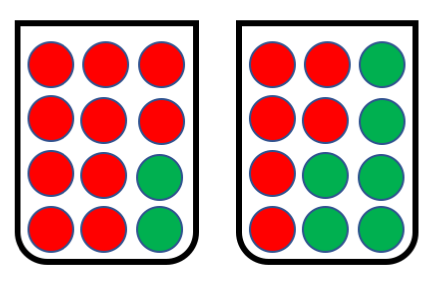## Classification impurity functions¶

• For classification: let $p_{1},...p_{C}$ be class probabilities for objects in node $t$.
• Then impurity function $\phi(t)=\phi(p_{1},p_{2},...p_{C})$ should satisfy:

• $\phi$ is defined for $p_{j}\ge0$ and $\sum_{j}p_{j}=1$.
• $\phi$ attains maximum for $p_{j}=1/C,\,k=1,2,...C$ .
• $\phi$ attains minimum when $\exists j:\,p_{j}=1,\,p_{i}=0$ $\forall i\ne j$.
• $\phi$ is symmetric function of $p_{1},p_{2},...p_{C}$.

## Typical classification impurity functions}¶

• Gini criterion

• interpretation: probability to make mistake when predicting class randomly with class probabilities $[p(\omega_{1}|t),...p(\omega_{C}|t)]$: $$I(t)=\sum_{i}p(\omega_{i}|t)(1-p(\omega_{i}|t))=1-\sum_{i}[p(\omega_{i}|t)]^{2}$$
• Entropy

• interpretation: measure of uncertainty of random variable $$I(t)=-\sum_{i}p(\omega_{i}|t)\ln p(\omega_{i}|t)$$
• Classification error

• interpretation: frequency of errors when classifying with the most common class $$I(t)=1-\max_{i}p(\omega_{i}|t)$$
In :
plot_impurities()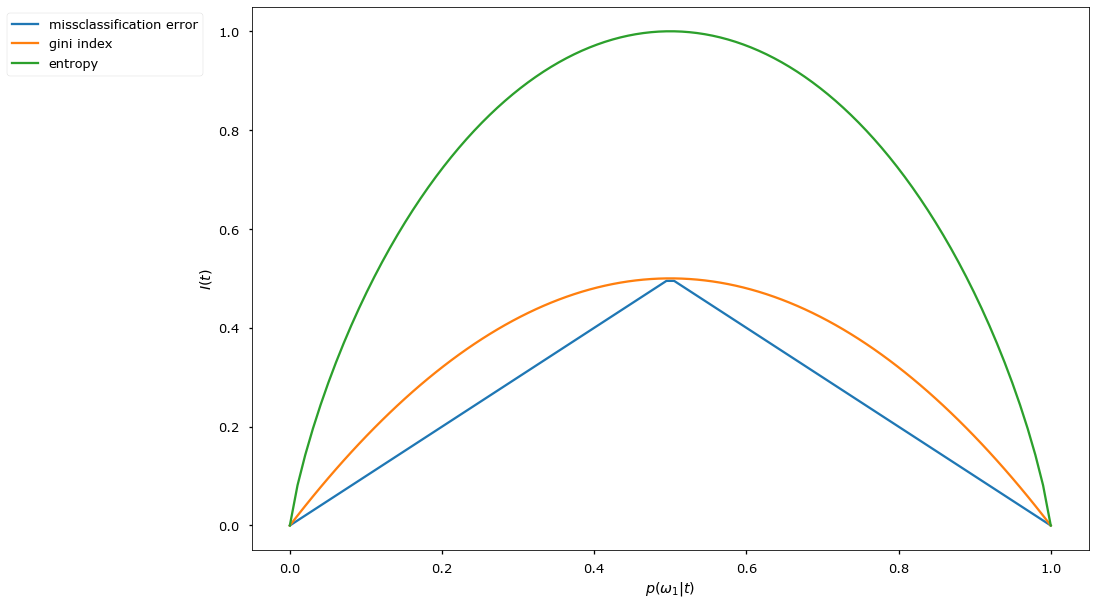## Splitting criterion selection¶

• Define $\Delta I(t)$ - the quality of the split of node $t$ into child nodes $t_{1},...t_{C}$. $$\Delta I(t)=I(t)-\sum_{i=1}^{C}I(t_{i})\frac{N(t_{i})}{N(t)}$$ $$\Delta I(t)=I(t)-\left(I(t_{L})\frac{N(t_{L})}{N(t)} + I(t_{R})\frac{N(t_{R})}{N(t)}\right)$$

• If $I(t)$ is entropy, then $\Delta I(t)$ is called information gain.
• CART optimization (regression, classification): select feature $i_{t}$ and threshold $h_{t}$, which maximize $\Delta I(t)$: $$i_{t},\,h_{t}=\arg\max_{k,h}\Delta I(t)$$
• CART decision making: from node $t$ follow:
$$\begin{cases} \text{left child }t_{1}, & \text{if }x^{i_{t}}\le h_{t}\\ \text{right child }t_{2}, & \text{if }x^{i_{t}}>h_{t} \end{cases}$$
In :
wine_demo()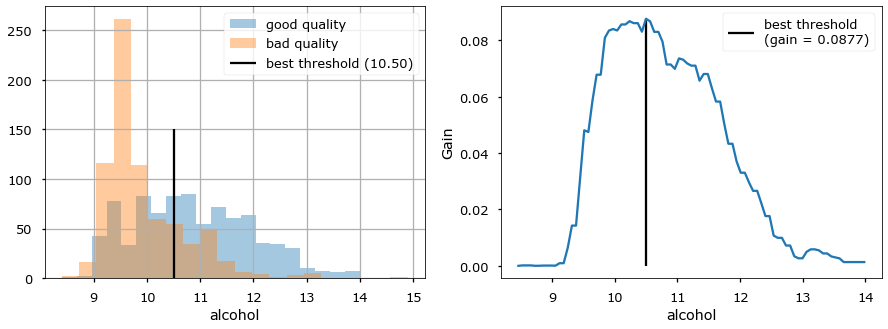## Typical regression impurity functions¶

• Impurity function measures uncertainty in $y$ for objects falling inside node $t$.
• Regression:
• let objects falling inside node $t$ be $I=\{i_{1},...i_{K}\}$. We may define \begin{align*} \phi(t) & =\frac{1}{K}\sum_{i\in I}\left(y_{i}-\mu\right)^{2}\quad \text{(MSE)}\\ \phi(t) & =\frac{1}{K}\sum_{i\in I}|y_{i}-\mu|\quad \text{(MAE)} \end{align*} where $\mu$ is mean or median of $y_i$s.

## Prediction assignment to leaves¶

• Regression:
• mean (optimal for MSE loss)
• median (optimal for MAE loss)
• Classification
• most common class (optimal for constant misclassification cost)

## Classification example¶

In :
fig = interact(demo_dec_tree, depth=IntSlider(min=1, max=5, value=1))


## Regression example¶

In :
fig = interact(plot_dec_reg, depth=IntSlider(min=1, max=5, value=1), criterion=['mse', 'mae'])


## Splitting criterion selection¶

Remarks

• Local and Greedy optimization
• Overall results changes slighly with different impurity measures
In :
plt.scatter(X_[:, 0], X_[:, 1], c=y_, cmap=plt.cm.Paired)

Out:
<matplotlib.collections.PathCollection at 0x1238fbe80>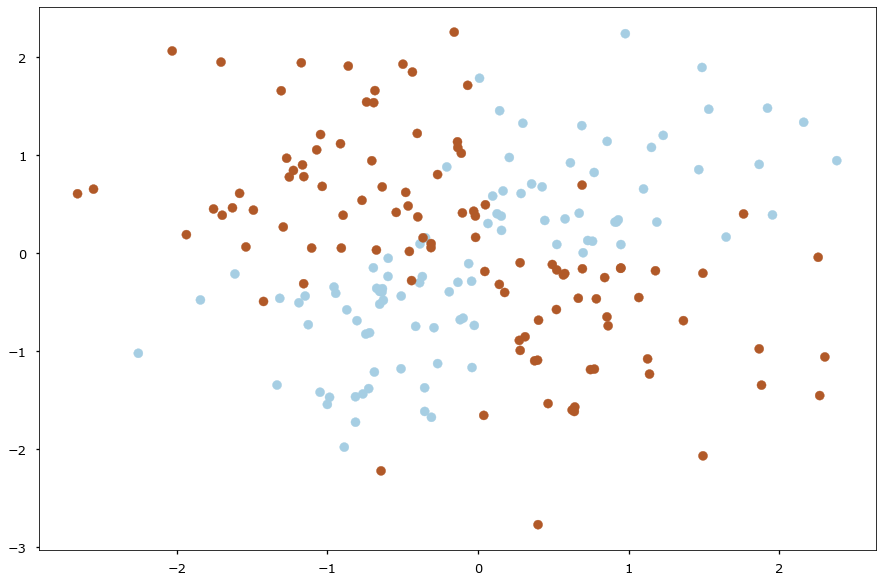In :
fig = interact(demo_dec_tree_xor, depth=IntSlider(min=1, max=6, value=1))


# Termination criterion¶

## Termination criterion¶

• very large complex trees -> overfitting
• very short simple trees -> underfitting
• Approaches to stop DC construction:
• rule-based stopping criterion
• based on pruning (not considered here)

## Rule-based termination criteria¶

• Rule-based: a criterion is compared with a threshold.
• Variants of criterion:
• depth of tree
• number of objects in a node
• minimal number of objects in one of the child nodes
• impurity of classes
• change of impurity of classes after the split
• etc

• simplicity
• interpretability

• specification of threshold is needed

## CART Cost-Complexity Prunning¶

• General idea: build tree up to pure nodes and then prune.
• Define:
• $T$ be some subtree of our tree
• $T_t$ full subtree with root at node $t$
• $\tilde{T}$ be a set of leaf nodes of tree $T$
• $R(t)$ - error measure inside node $t$ (#misclassifications, sum of squared errors)

Error rate on tree: $$R(T) = \sum\limits_{\tau \in \tilde{T}} R(\tau)$$

Error rate + complexity: $$R_\alpha(T) = \sum\limits_{\tau \in \tilde{T}} R(\tau)+ \alpha |T|$$

• Generally $R(T_t) < R(t)$, however if we consider $R_\alpha(\cdot)$...
• We can find $\alpha$ such that $R_\alpha(T_t) = R_\alpha(t)$ $$\alpha_t = \frac{R(t) - R(T_t)}{|\tilde{T_t}| - 1}$$

## The algorithm¶

1. Build the most "puriest" tree $T_0$ that and set $\alpha_0 = 0$, $i=0$
2. Until the tree is completely prunned do:
• i++
• find node $t$ that minimizes $$\alpha_i = \frac{R(t) - R(T_t)}{|\tilde{T_t}| - 1}$$
• Replace $T_t$ with $t$

Output:

• sequence of $\alpha_0 \leq \alpha_1 \leq \dots \leq \alpha_K$
• with correspondent prunned tries $T_0 \supseteq T_1 \supseteq \dots \supseteq T_K$
• choose $T_i$ with lowest error on validation set
In :
fig = interact(plot_dec_reg_alpha, alpha=FloatSlider(min=0, max=0.05, value=0, step=0.0005, readout_format='.4f'))


# Other features¶

## Tree feature importances¶

• Consider feature $f$
• Let $T(f)$ be the set of all nodes, relying on feature $f$ when making split.
• efficiency of split at node $t$: $\Delta I(t)=I(t)-\sum_{c\in childen(t)}\frac{n_{c}}{n_{t}}I(c)$
• feature importance of $f$: $\sum_{t\in T(f)}n_{t}\Delta I(t)$

## Handling missing values¶

1. Remove features or objects with missing values
2. Missing value = distinct feature value
3. Calculation of impurity w/o missing cases
4. Surrogate split!
• Find best split with feature $i^*$, threshold $h^*$ and children $\{t^*_L, t^*_R\}$
• Find other good splits for features $i_t \neq i^*$, s.t. $\{t_L, t_R\} \approx \{t^*_L, t^*_R\}$
• While performing prediction of object $x$:
• If $x^{i^*}$ is Null, try $x^{i_t}$

## Analysis of decision trees¶

• simplicity of algorithm
• interpretability of model (for short trees)
• implicit feature selection
• good for features of different nature:
• naturally handles both discrete and real features
• prediction is invariant to monotone transformations of features

## Analysis of decision trees¶

• not very high accuracy:
• high overfitting of tree structure
• non-parallel to axes class separating boundary may lead to many nodes in the tree for $Q_{t}(x)=x^{i(t)}$
• one step ahead lookup strategy for split selection may be insufficient (XOR example)
• not online - slight modification of the training set will require full tree reconstruction.

## Special Desicion Tree Algorithms¶

ID 3

• Categorical features only
• Number of children = number of categories
• Maximum depth

С 4.5

• Handling continious features
• And categorical as in ID3
• Find missing value - proceed down to all paths and average
• Some prunning procedure

## Interpretation of DT¶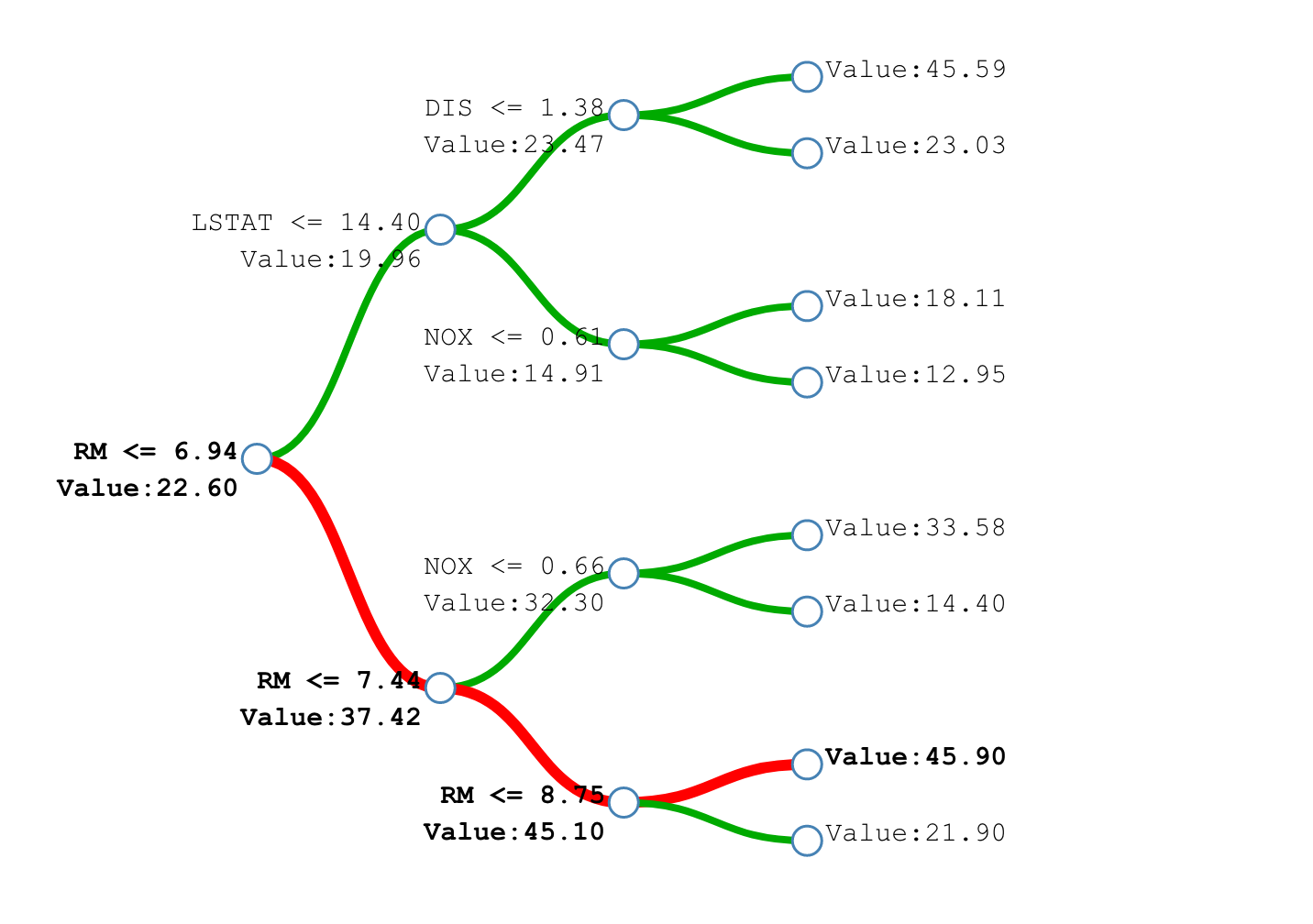• How tree works
• Mohammed J. Zaki, et al: Data Mining and Analysis - Fundamental Concepts and Algorithms - Chapter 19
• Andrew R. Webb, et al: Statistical Pattern Recognition - Chapter 7
• L. Breiman, J. Friedman, R. Olshen, and C. Stone. Classification and Regression Trees. Wadsworth, Belmont, CA, 1984.
• Cost-complexity prunning in sklearn + example